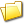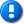home | career | drupal | java | mac | mysql | perl | scala | uml | unix

# Java example source code file (RandomStraightLinePointGenerator.java)

This example Java source code file (RandomStraightLinePointGenerator.java) is included in the alvinalexander.com "Java Source Code Warehouse" project. The intent of this project is to help you "Learn Java by Example" TM.

## Java - Java tags/keywords

awt, deprecated, geometry, normaldistribution, randomgenerator, randomstraightlinepointgenerator, realdistribution, uniformrealdistribution, well44497b

## The RandomStraightLinePointGenerator.java Java example source code

```/*
* Licensed to the Apache Software Foundation (ASF) under one or more
* contributor license agreements.  See the NOTICE file distributed with
* The ASF licenses this file to You under the Apache License, Version 2.0
* (the "License"); you may not use this file except in compliance with
* the License.  You may obtain a copy of the License at
*
*
* Unless required by applicable law or agreed to in writing, software
* WITHOUT WARRANTIES OR CONDITIONS OF ANY KIND, either express or implied.
* See the License for the specific language governing permissions and
*/

package org.apache.commons.math3.optimization.general;

import java.awt.geom.Point2D;

import org.apache.commons.math3.random.RandomGenerator;
import org.apache.commons.math3.random.Well44497b;
import org.apache.commons.math3.distribution.RealDistribution;
import org.apache.commons.math3.distribution.UniformRealDistribution;
import org.apache.commons.math3.distribution.NormalDistribution;

/**
* Factory for generating a cloud of points that approximate a straight line.
*/
@Deprecated
public class RandomStraightLinePointGenerator {
/** Slope. */
private final double slope;
/** Intercept. */
private final double intercept;
/** RNG for the x-coordinate. */
private final RealDistribution x;
/** RNG for the error on the y-coordinate. */
private final RealDistribution error;

/**
* The generator will create a cloud of points whose x-coordinates
* will be randomly sampled between {@code xLo} and {@code xHi}, and
* the corresponding y-coordinates will be computed as
* <pre>```
*  y = a x + b + N(0, error)
* </code>``````
``` * where {@code N(mean, sigma)} is a Gaussian distribution with the * given mean and standard deviation. * * @param a Slope. * @param b Intercept. * @param sigma Standard deviation on the y-coordinate of the point. * @param lo Lowest value of the x-coordinate. * @param hi Highest value of the x-coordinate. * @param seed RNG seed. */ public RandomStraightLinePointGenerator(double a, double b, double sigma, double lo, double hi, long seed) { final RandomGenerator rng = new Well44497b(seed); slope = a; intercept = b; error = new NormalDistribution(rng, 0, sigma, NormalDistribution.DEFAULT_INVERSE_ABSOLUTE_ACCURACY); x = new UniformRealDistribution(rng, lo, hi, UniformRealDistribution.DEFAULT_INVERSE_ABSOLUTE_ACCURACY); } /** * Point generator. * * @param n Number of points to create. * @return the cloud of {@code n} points. */ public Point2D.Double[] generate(int n) { final Point2D.Double[] cloud = new Point2D.Double[n]; for (int i = 0; i < n; i++) { cloud[i] = create(); } return cloud; } /** * Create one point. * * @return a point. */ private Point2D.Double create() { final double abscissa = x.sample(); final double yModel = slope * abscissa + intercept; final double ordinate = yModel + error.sample(); return new Point2D.Double(abscissa, ordinate); } } Other Java examples (source code examples) Here is a short list of links related to this Java RandomStraightLinePointGenerator.java source code file:The search pageOther Java source code examples at this package levelClick here to learn more about this project ```